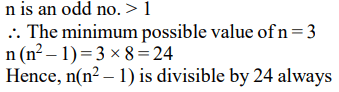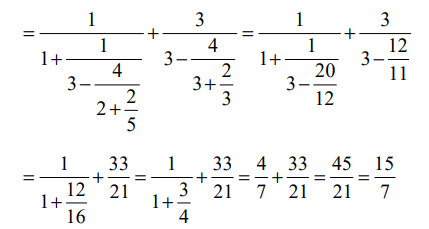## Previous Year CAT Questions on Number System

1. If n is any odd number greater than 1, then $n\left(n^{2}-1\right)$  is
a) divisible by 48 always
b) divisible by 24 always
c) divisible by 6 always
d) None of these

Explanation:2. Find the value of $\frac{1}{1+\frac{1}{3-\frac{4}{2+\frac{1}{3-\frac{1}{2}}}}}+\frac{3}{3-\frac{4}{3+\frac{1}{2-\frac{1}{2}}}}$
a) 13/7
b) 15/7
c) 11/21
d) 17/28

Explanation:3. If n is an integer, how many values of n will give an integral value of $\left(16n^{2}+7n+6\right)$   / n ?
a) 2
b) 3
c) 4
d) None of these

Explanation:4. A student, instead of finding the value of 7/8th of a number, found the value of 7/18th of the number. If his answer differed from the actual one by 770, find the number.
a) 1584
b) 2520
c) 1728
d) 1656

Explanation:5. If m and n are integers divisible by 5, which of the following is not necessarily true?
a) m – n is divisible by 5
b) $m^{2}+n^{2}$  is divisible by 25
c) m + n is divisible by 10
d) None of these

Explanation: If m & n are integers divisible by 5.
Then, (m + n) might be or might not be divisible by 10.
For example: If m = 5 and n = 10 then m + n = 15 which is not divisible by 10.
But if m = 5, n = 25 then m + n = 30 which is divisible by 10

6. Which of the following is true?
a) $7^{3^{2}}=\left(7^{3}\right)^{2}$
b) $7^{3^{2}}>\left(7^{3}\right)^{2}$
c) $7^{3^{2}}<\left(7^{3}\right)^{2}$
d) None of these

Explanation:7. P, Q and R are three consecutive odd numbers in ascending order. If the value of three times P is three less than two times R, find the value of R.
a) 5
b) 7
c) 9
d) 11

Explanation: Let P, Q and R be n, n + 2 and n + 4 respectively in ascending order.
According to the Question
3n = 2(n + 4) – 3 = 2n + 5
n = 5
Thus, R = 5 + 4 = 9

8. A, B and C are defined as follows :
$A=\left(2.000004\right)\div\left[\left(2.000004\right)^{2}+\left(4.000008\right)\right]$
$B=\left(3.000003\right)\div\left[\left(3.000003\right)^{2}+\left(9.000009\right)\right]$
$C=\left(4.000002\right)\div\left[\left(4.000002\right)^{2}+\left(8.000004\right)\right]$
Which of the following is true about the value of the above three expressions?
a) All of them lie between 0.18 and 0.20
b) A is twice of C
c) C is the smallest
d) B is the smallest

Explanation:9. P and Q are two integers such that (PQ) = 64. Which of the following cannot be the value of P + Q?
a) 20
b) 65
c) 16
d) 35

Explanation: Given PQ = 64 = 1 × 64 = 2 × 32 = 4 × 16 = 8 × 8.
Corresponding values of P + Q are 65, 34, 20, 16.
Therefore, P + Q cannot be equal to 35.

10. Five digit numbers are formed using only 0,1,2,3,4 exactly once. What is the difference between the maximum and minimum number that can be formed?
a) 19800
b) 41976
c) 32976
d) None of these